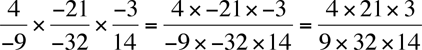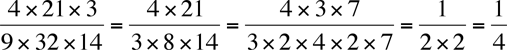Quandaries and Queries How to simplify following Grade 9 math problem: (4/-9) X (-21/-32) X (-3/14) Thanks, Vijay Hi Vijay, The first thing to realize is that you can multiply the numerators to get one numerator and multiply the denominators to get one denominator. That isNotice that there are an even number of minus signs in each of the numerator and denominator so both are positive. Now look for some cancellation. 33 = 9 and 48 = 32 soPenny